Practice Test: Civil Engineering (CE)- 13

# Practice Test: Civil Engineering (CE)- 13 - Civil Engineering (CE)

Test Description

## 65 Questions MCQ Test GATE Civil Engineering (CE) 2023 Mock Test Series - Practice Test: Civil Engineering (CE)- 13

Practice Test: Civil Engineering (CE)- 13 for Civil Engineering (CE) 2023 is part of GATE Civil Engineering (CE) 2023 Mock Test Series preparation. The Practice Test: Civil Engineering (CE)- 13 questions and answers have been prepared according to the Civil Engineering (CE) exam syllabus.The Practice Test: Civil Engineering (CE)- 13 MCQs are made for Civil Engineering (CE) 2023 Exam. Find important definitions, questions, notes, meanings, examples, exercises, MCQs and online tests for Practice Test: Civil Engineering (CE)- 13 below.
Solutions of Practice Test: Civil Engineering (CE)- 13 questions in English are available as part of our GATE Civil Engineering (CE) 2023 Mock Test Series for Civil Engineering (CE) & Practice Test: Civil Engineering (CE)- 13 solutions in Hindi for GATE Civil Engineering (CE) 2023 Mock Test Series course. Download more important topics, notes, lectures and mock test series for Civil Engineering (CE) Exam by signing up for free. Attempt Practice Test: Civil Engineering (CE)- 13 | 65 questions in 180 minutes | Mock test for Civil Engineering (CE) preparation | Free important questions MCQ to study GATE Civil Engineering (CE) 2023 Mock Test Series for Civil Engineering (CE) Exam | Download free PDF with solutions
 1 Crore+ students have signed up on EduRev. Have you?
Practice Test: Civil Engineering (CE)- 13 - Question 1

### A monkey is trying to reach the top of a tree. He climbs 3 feet in a minute but slides down by 1.5 feet after each climb. If he reaches the top of the tree in 58 minutes, then the maximum possible height of the tree is ________ feets.

Detailed Solution for Practice Test: Civil Engineering (CE)- 13 - Question 1
You have to carefully observe the point that at the last minute, we DO NOT have to consider the sliding down of the monkey since the question states that the monkey reaches the top of the tree. Out of 58 minutes, the climb by the monkey in the 58th minute is 3 feet whereas for each of his earlier climbs, the monkey covers a net distance of 3 – 1.5 = 1.5 feet.

We can work out the maximum possible height of the tree as 1.5 x 57 + 3 = 85.5 + 3 = 88.5 feet

Practice Test: Civil Engineering (CE)- 13 - Question 2

### In the following question, a sentence has been given in Direct/Indirect Speech. Out of the four alternatives suggested, select the one which best expresses the same sentence in Indirect/Direct Speech.My husband said to me, “Wait for me outside."

Detailed Solution for Practice Test: Civil Engineering (CE)- 13 - Question 2
This is an imperative sentence as there is instruction being given by the husband to his wife. Since the reported speech is not signifying the mode of behaviour here whether it was a request, a command or was it said in anger, we cannot use verbs related to such emotions. We'll simply convert "said to" into "told". In affirmative imperative sentences, we use "to" for connecting reporting and reported speech. The correct answer is option A as it follows the above rules correctly.
Practice Test: Civil Engineering (CE)- 13 - Question 3

### What of the following function(s) in an accurate description of the graph for the range(s) indicated?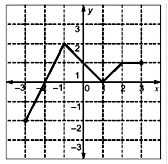A. y = 2x + 4 for -3 ≤ x ≤ -1B. y = |x-1| for -1 ≤ x ≤ 2C. y = ||x|-1| for -1 ≤ x ≤ 2D. y = 1 for 2 ≤ x ≤ 3

Detailed Solution for Practice Test: Civil Engineering (CE)- 13 - Question 3
Put value and verify

(i) y = 2x+4 is true in -3 ≤ x ≤ -1

On putting x = -3, y = 2 and x = -2,y = 0 and x = -1, y = 2

(ii) y = |x-1| is also true (x = -1, y = 2), (x = 0 ,y = 1) and (x = 1, y = 0)

(iv) y = 1 in (2 ≤ x ≤ 3) always true

(i), (ii) and (iv) are true.

Practice Test: Civil Engineering (CE)- 13 - Question 4

A rectangular room having dimensions 3.74 m x 5.78 m is required to be tiled using a minimum number of identical square tiles. The number of such tiles and area of each tile (in cm2) is given by

Detailed Solution for Practice Test: Civil Engineering (CE)- 13 - Question 4
In this question, we need to find the HCF of 374 and 578 which is 34. We can look at 374 = 34 x 11 and 578 = 34 x 17. This gives the number of square tiles required to cover the entire room is 11 x 17 = 187 and the area of each such tile is 342 = 1156 cm2.
Practice Test: Civil Engineering (CE)- 13 - Question 5

In the following question, out of the four alternatives, select the alternative which best expresses the meaning of the Idiom/Phrase.

To take into account

Detailed Solution for Practice Test: Civil Engineering (CE)- 13 - Question 5
The phrase "take into account" means to consider or remember something when judging a situation. Thus, option A is the correct answer.
Practice Test: Civil Engineering (CE)- 13 - Question 6

In an examination, a candidate attempts all the questions since there is no negative marking and all the questions carry equal marks. Out of the first 30 questions that he attempts, he has marked correct options for 20 questions whereas out of the remaining questions, he is able to mark only one third question with correct option. His overall score in the examination is 50%.

The total number of questions in the paper are;

Detailed Solution for Practice Test: Civil Engineering (CE)- 13 - Question 6

We can form equations based on the given information. Let ‘x’ be the number of questions attempted by him in the second part.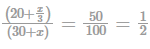Or 40+ 2x/3 = 30 + x which leads us to get x/3 = 10 or x = 30

The total number of questions in the examination are x + 30 = 60

Practice Test: Civil Engineering (CE)- 13 - Question 7

Amol, Brijendra, Chander and Dron are sitting around a roundtable. One of them is an electronics engineer (ECE) who is sitting to the left of the Mechanical Engineer (ME). Amol is sitting opposite a Computer Science Engineer (CSE). It is also known that Dron likes to play computer games and Brijendra is sitting to the right of Civil Engineer (CE). Identify the correct match from the given choices.

Detailed Solution for Practice Test: Civil Engineering (CE)- 13 - Question 7

Based on the given data, we can say that Amol (ME) is sitting opposite to CSE – Brijendra. Civil Engineer has to be to the right of Amol – Mechanical Engineer. However, it is not possible to say whether Chander is an Electronics Engineer or a Civil Engineer AND likewise for Dron.

Practice Test: Civil Engineering (CE)- 13 - Question 8

In five cities A is more populated than B which is less populated then E. D is more populated than E but not as populated as A. C is less populated than B. Which city has the highest population?

Detailed Solution for Practice Test: Civil Engineering (CE)- 13 - Question 8

From first statement

A > B and B < E

From second statement

D > E and D < A

From third statement

C < B

From all statements together

A > D > E > B > C

Practice Test: Civil Engineering (CE)- 13 - Question 9

One-third of Ramesh’s marks in Arithmetic is equal to half his marks in English. If he gets 150 marks in the two subjects to gather, how many marks has he got in English?

Detailed Solution for Practice Test: Civil Engineering (CE)- 13 - Question 9

Let marks obtained by Ramesh in Arithmetic be ‘A’ and marks obtained in English be E.

As the information given,

1/3 A = E/2

Ã (A/3) – (E/2) = 0

Ã 2A – 3E= 0 ……….(i)

A + E = 150 …………(ii)

From equation (i) and (ii), E = 60

Hence, Ramesh got 60 marks in English.

Practice Test: Civil Engineering (CE)- 13 - Question 10

In a test given by Prof Virus during the minors, Raju gets 32% marks and fails by 20 marks whereas Chatur gets 30 marks more than passing marks while scoring 42% marks. The marks required to get twice the passing marks are _______.

Detailed Solution for Practice Test: Civil Engineering (CE)- 13 - Question 10

The difference in percentage marks obtained by Raju and Chatur is 42 – 32 = 10% whereas the difference in their marks is 20 + 30 = 50 (Raju gets 20 marks less while Chatur gets 30 marks more than pass marks).

This gives us 1% = 5 marks and the passing marks as 20 + 32% = 180.

The required marks = 360 (twice the passing marks)

Practice Test: Civil Engineering (CE)- 13 - Question 11

During the subsurface investigations for design of foundations, a standard penetration test was conducted at 4.5m below the ground surface. The record of number of blows is given below.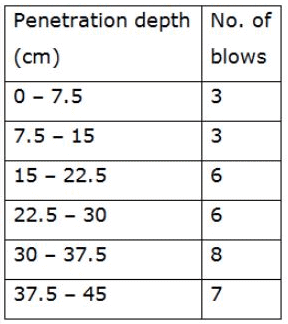Q. Assuming the water table at ground level, soil as fine sand and correction factor for overburden as 1.0, the corrected ‘N’ value for the soil would be

Detailed Solution for Practice Test: Civil Engineering (CE)- 13 - Question 11
Standard penetration test – number of penetration for the first 15 cm is not counted and only the number of blows which causes further 30 cm of penetration is taken into account.

So, here N = 6 + 6 + 8 + 7 = 27

Now, here N > 15 and water table at ground level so dilatancy correction is applied.

Ncorrected = 15 + 1/2(N−15)

= 15 + 1/2(27 - 15) = 21

*Answer can only contain numeric values
Practice Test: Civil Engineering (CE)- 13 - Question 12

A beam-column having overall depth and width is 550 mm and 250 mm. If torsional moment for this beam is 30 kN.m then twisting moment will be-

Detailed Solution for Practice Test: Civil Engineering (CE)- 13 - Question 12
Given, b = 250 mm, D = 550 mm

& T = 30 kN .m

So, the twisting moment

MT = T (1 + D/b) / 1.7

= 30 (1 + 550/250) / 1.7

= 56.47 kN .m

Practice Test: Civil Engineering (CE)- 13 - Question 13

An isolated ‘T’ beam is used on the walkway. The beam is simply supported with an effective span of 6 m. Effective width of flange for shown figure is :

Detailed Solution for Practice Test: Civil Engineering (CE)- 13 - Question 13
Effective width for isolated T beam is given as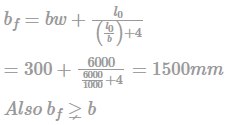Hence bf = 1000 mm

Practice Test: Civil Engineering (CE)- 13 - Question 14

What is the maximum possible eccentricity in a prestressed concrete beam of circular cross-section? Diameter of the section is d. Tension is not allowed anywhere and any time in the cross-section. Neglect dead load (self-weight).

Detailed Solution for Practice Test: Civil Engineering (CE)- 13 - Question 14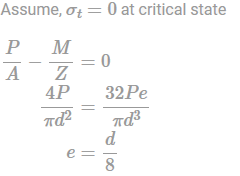Practice Test: Civil Engineering (CE)- 13 - Question 15

Deposit with flocculated structure is formed when

Detailed Solution for Practice Test: Civil Engineering (CE)- 13 - Question 15
• The process by which individual particles of clay aggregate into coltlike masses or precipitate into small lumps. Flocculation occurs as a result of a chemical reaction between the clay particles and another substance, usually salt water.

• In the case of flocculated structure, there will be edge to edge and edge to face contact between the particles. This type of formation is due to the net electrical forces between the adjacent particles at the time of deposition being attractive in nature.

• The concentration of dissolved minerals in water leads to formation of flocculated structures with very high void ratio as in the case of marine deposits.

*Answer can only contain numeric values
Practice Test: Civil Engineering (CE)- 13 - Question 16

A simply supported prestressed beam of 300 × 500 mm in c/s is subjected to a superimposed load of 20 kN/m over a span of 10m. If a prestressing force of 1000 kN is applied through a straight tendon located along centroidal axis then what is the extreme top fiber stress at the mid section:

Detailed Solution for Practice Test: Civil Engineering (CE)- 13 - Question 16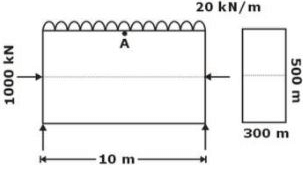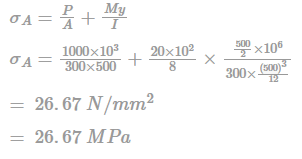Practice Test: Civil Engineering (CE)- 13 - Question 17

What will be the maximum possible uniformly distributed load (inclusive of self-weight) over the entire span of a simply supported beam of span ‘L’ such that the deflection at midspan at service condition is zero? The cross-section is rectangular. The prestressing force ‘P’ is applied with uniform eccentricity ‘e’. Assume no losses.

Detailed Solution for Practice Test: Civil Engineering (CE)- 13 - Question 17
For no deflection at midspan,

[δ due to self (weight + udl)] + [δ due to eccentric force P on cable] = 0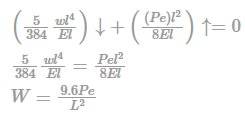Practice Test: Civil Engineering (CE)- 13 - Question 18

If Qs4 and Qs6 are the equilibrium discharges of S- curve for the catchment obtained by 4 hour UH summation and 6 hour UH summation, respectively, then Qs4/Qs6 is __________. (Up to 2 decimal places)

Detailed Solution for Practice Test: Civil Engineering (CE)- 13 - Question 18

Qe = A.1D

Qs4/Qs6 = 6/4 = 1.50

*Answer can only contain numeric values
Practice Test: Civil Engineering (CE)- 13 - Question 19

The beam PQR shown in the given figure is horizontal. The distance to the point of contraflexure from the fixed end P is _____m. (up to two decimal place)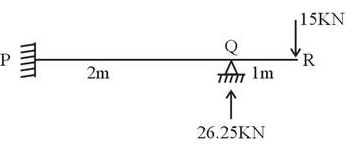Detailed Solution for Practice Test: Civil Engineering (CE)- 13 - Question 19
Let the point of contraflexure be at a distance ′x′ from the free end.

15x = 26.25(x−1)

x = 2.334m

→ Distance from fixed end = 3 - 2.334 = 00.666m.

Practice Test: Civil Engineering (CE)- 13 - Question 20

30 KN/m2 load acting on a 4m × 6m 2-way slab. Effective Load acting in a shorter direction is_____ KN/m2.

Detailed Solution for Practice Test: Civil Engineering (CE)- 13 - Question 20
wx = Load acting in shorter direction

wy = Load acting in shorter direction

wx + wy = w

Equating deflection of both strips.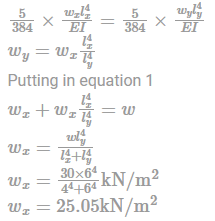Practice Test: Civil Engineering (CE)- 13 - Question 21

A stream function is given by:

Ψ = 3xy2 + (x2+2)y

The flow rate across a line joining points A(0,5) and B(1,2) is

Detailed Solution for Practice Test: Civil Engineering (CE)- 13 - Question 21
ΨA = (3 × 0 × 52) + ((02 + 2)×5) = 10

ΨB = (3×1×22) + ((12 + 2) × 2) = 18

Flow rate across a line joining points A and B is

= ΨB − ΨA

= 18 − 10

= 8 units.

Practice Test: Civil Engineering (CE)- 13 - Question 22

Before passage of a surge, the depth and velocity of flow at a section are 1.8 m and 3.6 m/s. After the surge passage, they are 0.6 m and 8 m/s respectively. The speed of the surge is?

Detailed Solution for Practice Test: Civil Engineering (CE)- 13 - Question 22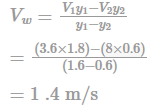Practice Test: Civil Engineering (CE)- 13 - Question 23

A rectangular plate 1.2 x 3 m is immersed in a liquid of relative density 0.85 with its 1.2 m side horizontal and just at the water surface. If the plane of plate makes an angle of 50o with the horizontal, the pressure force on one side of the plate is

Detailed Solution for Practice Test: Civil Engineering (CE)- 13 - Question 23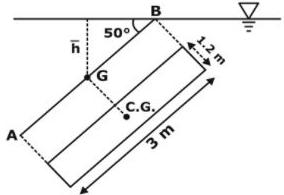Density of liquid = 0.85 x 1000 = 850 kg/m3

h = 1.5 sin 50o = 1.149 m

Pressure force on one side of plate = ρgAh = 850 x 9.81 x (1.2 x 3) x 1.149 = 34.5 kN.

Practice Test: Civil Engineering (CE)- 13 - Question 24

A beam with both continuous ends has a clear span of 6m and supports 600 mm wide. The effective depth of the beam is 550 mm. As per IS 456-2000, the effective span of the beam (in m) is____________

Detailed Solution for Practice Test: Civil Engineering (CE)- 13 - Question 24
As per IS 456 —2000, the effective span of continuous beam

i) When width of support < 1/12="" of="" clear="" />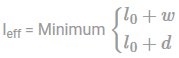ii) when width of support > 1/12 of clear span

a) When one end is fixed & other end continuous or both ends continuous.

leff = lo

b) When one end is continuous & the other end is simply supported.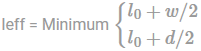As per given data, width of support = 600 mm & 1/12 of clear span = 500 mm

Hence leff = lo = 6m [since both ends are continuous]

Practice Test: Civil Engineering (CE)- 13 - Question 25

A turbine develops 2516 kW at 240 rpm. The torque in the shaft is approximately:

Detailed Solution for Practice Test: Civil Engineering (CE)- 13 - Question 25
As we know P = (2πNT)/60

Where, T = torque and N = speed in rpm

So, T = 60P/(2πN) = (60×2516×1000)/(2×3.14×240) = 100108Nm = 100kNm

Practice Test: Civil Engineering (CE)- 13 - Question 26

A Kaplan turbine has a runner of diameter 4.0 m. The diameter of the hub is 1.6 m. If the velocity of flow and the swirl velocity at the inlet side of the blade at the hub are 6.0 m/s and 10.0 m/s, respectively, the flow and swirl velocities at the inlet side of the tip are, respectively:

Detailed Solution for Practice Test: Civil Engineering (CE)- 13 - Question 26
Since the area of flow remains the same.

The flow velocity remains the same throughout.

Hence Vf2 = Vf1 = 6 m/s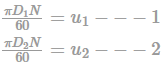D1 = 1.6; D2 = 4; u2 = 10m/s

Hence u1 = 4 m/s

Practice Test: Civil Engineering (CE)- 13 - Question 27

The plastic moment at collapse is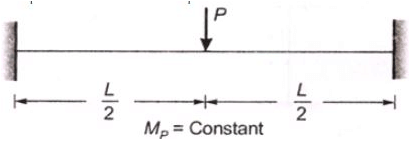Detailed Solution for Practice Test: Civil Engineering (CE)- 13 - Question 27
Number of plastic hinges needed are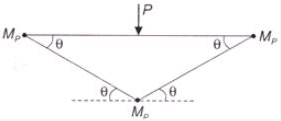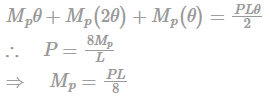Practice Test: Civil Engineering (CE)- 13 - Question 28

A pipe of 30 cm diameter conveying 300 l/s of water has a right-angled bend in a horizontal plane. The resultant force exerted on the bend if pressure at inlet and outlet of the bend are 24.525N/cm2 and 23.544N/cm2 is ___ N.

Detailed Solution for Practice Test: Civil Engineering (CE)- 13 - Question 28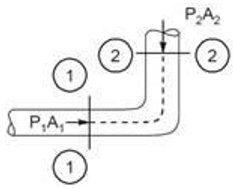D = 30 cm

A1 = A2 = π/4D2 = 0.07068 m2

V = V1 = V2 = Q/A = 0.3/0.07068 = 4.244 m/s

Now, θ = 90°,

P1 = 24.525 N/cm2 = 24.525 × 104 N/m2

P2 = 23.544 × 104 N/m2

Force along x-axis on bend,

LFx = pQ[V1x - V2x]+(P1xA1) + P2xA2

Fx = 1000 × 0.3[4.244-0] + 24.525 × 104 × 0.07068 + 0

Fx = 18607.5N Similarly, force along y-axis on bend,

Fy = pQ [V1y - V2y] + P1yA1 + P2yA2

Fy = 1000 × 0.3 [0- 4.244 ] + 0 - 235440 × 0.07068

Fy = 17914.1 N

FR =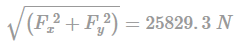Practice Test: Civil Engineering (CE)- 13 - Question 29

A sample of saturated cohesionless soil tested in a drained triaxial compression test showed an angle of internal friction of 30°. The deviator stress at failure for the sample at a confining pressure of 200kPa is equal to

Detailed Solution for Practice Test: Civil Engineering (CE)- 13 - Question 29
σ1 = σ3tan2α

Where, α = 45 + ϕ/2

α = 45∘ + 30/2 = 60, σ1 = 3σ3 = 3 × 200 = 600 kPa

∴ Deviatoric stress = σ1 - σ3

= 600 - 200 = 400 kPα

Practice Test: Civil Engineering (CE)- 13 - Question 30

The daily cover of MSW landfills consists of which one of the following?

Detailed Solution for Practice Test: Civil Engineering (CE)- 13 - Question 30
The municipal solid waste (MSW) is simply brought and dumped on the ground, spread in layers of about 0.5 m thickness, and compacted Another layer of 0.5 m thickness is then placed on top of the previous layer and also compacted Layering and compacting are repeated until a height of about 1.5 m is reached. At this point and at the end of a working day, a cover of earth of about 0.15 to 0.3 m thickness is compacted on the top and side slopes of the compacted heap, which is called a cell. This cover is called the daily cover.
Practice Test: Civil Engineering (CE)- 13 - Question 31

Two different soil types (soil I and Soil 2) are used as backfill behind a retaining wall as shown in the figure, where gt is total unit weight, and c ¢ and f ¢ are effective cohesion and effective angle of shearing resistance. The resultant active earth force per unit length (in kN/m) acting on the wall is: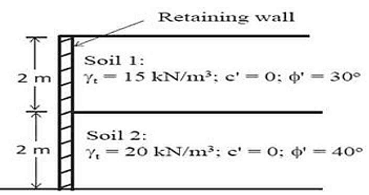Detailed Solution for Practice Test: Civil Engineering (CE)- 13 - Question 31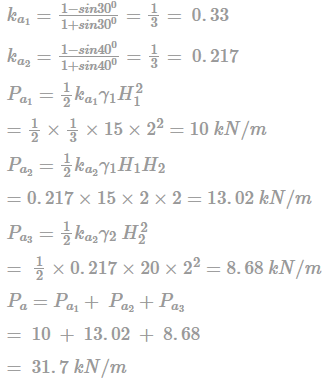Practice Test: Civil Engineering (CE)- 13 - Question 32

What is the final end moment in the following structure?

Detailed Solution for Practice Test: Civil Engineering (CE)- 13 - Question 32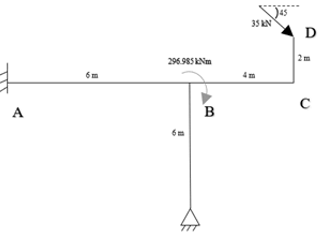Moment at the joint,

= 35 cos 45 × 2 + 35 sin 45 × 4

= 148.5 kNm

Stiffness of AB = 4EI/6

Stiffness of BC = 3EI/6

Distribution factor of AB,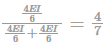Moment transferred to AB,

MBA = (4/7) × 148.5 = 84.86 kNm

Fixed end moment

MAB = (1/2) × 84.86 = 42.43 kNm

Practice Test: Civil Engineering (CE)- 13 - Question 33

In an irrigation project, in a certain year, 70% and 46% of the culturable command area in Kharif and Rabi, respectively, remained without water and the rest of the area got irrigation water. The intensity of irrigation in that year for the project was

Detailed Solution for Practice Test: Civil Engineering (CE)- 13 - Question 33
The annual intensity of irrigation is the sum of total intensities of irrigation of all the seasons of the year

= Intensity in Rabi + Intensity in Kharif

= (100-46) + (100-70)

= 84%

Practice Test: Civil Engineering (CE)- 13 - Question 34

A retaining wall is constructed in a clayey soil. The height of the backfill is found to be 9m, the unit weight of the sand is 2t/m3 and the angle of internal friction is 30˚. Find out the earth pressure per meter length on the retaining wall with a smooth vertical back.

Detailed Solution for Practice Test: Civil Engineering (CE)- 13 - Question 34
Active pressure coefficient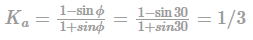Active earth pressure per unit length = 0.5 x Kα x y x H2 = 0.5 x (1/3) x 2t x 9 x 9 = 27t/m

Practice Test: Civil Engineering (CE)- 13 - Question 35

Activities A, B, C and D constitute a small project; their interrelationship, expected duration and standard deviation of this expected duration are shown in the figure, respectively.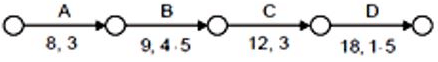With a view to improving the speed of implementation, each of B, C and D are split into three equal segments, maintaining appropriate inter-relationships between A and each of these nine segments. What will be the standard deviation of the modified project duration after segmentation (to the nearest 1/10in unit)?

Detailed Solution for Practice Test: Civil Engineering (CE)- 13 - Question 35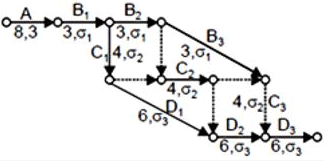If activity B is divided into 3 equal parts then each part will have duration 3 units and standard deviationσ1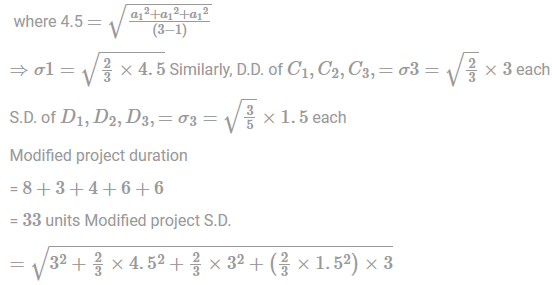= 5.6

Practice Test: Civil Engineering (CE)- 13 - Question 36

The infiltration capacity of a soil follows the Horton's exponential model, f = c. During and experiment, the initial infiltration capacity was observed to be 200 mm/h. After a long time, the infiltration capacity was reduced to 25 mm/h. If the infiltration capacity after 1 hour was 90 mm/h, the value of the decay rate constant, k (in 11', up to two decimal places) is____

Detailed Solution for Practice Test: Civil Engineering (CE)- 13 - Question 36

Horton's infiltration capacity

f = fc + (f0−fi)e−∞t

fε = ultimate infiltration capacity = 25 mm/hr

f = Initial infiltration capacity = 200 mm/hr

f = Infiltration capacity = 90 mm/hr

f = fc + (f0−fi)e−∞t

90 = 25 + (200 - 25) e-∞x1

175e-x = 65

E-x = 0.371

∝ = 0.9915; ∝ = 0.99/hou!

Practice Test: Civil Engineering (CE)- 13 - Question 37

Reciprocal leveling was done between two points A and B, the readings of which are as shown below, which are 700 mm apart.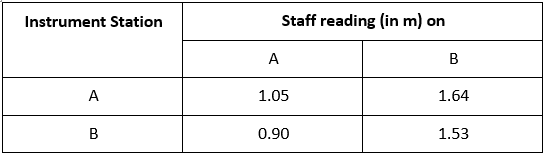If the atmospheric conditions do not change during the period of observation and R.L. of A is 126.49 m, then the R.L. of B is _______ m.

Detailed Solution for Practice Test: Civil Engineering (CE)- 13 - Question 37

True difference of level between A and B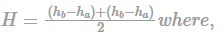hb = reading on staff at B when instrument at A = 1.64

ha = reading on staff at A when instrument at A = 1.05

hb = reading on staff at B when instrument at B = 1.53

ha = reading on staff at A when instrument at B = 0.90

⇒ H = 0.61m ∴ R.L. of B = R.L. of A-H = 125.88m

Note: R.L of B

Practice Test: Civil Engineering (CE)- 13 - Question 38

Let P be a 2 ×2 real orthogonal matrix and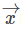is a real vector [x1, x2]T with length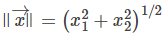Then, which one of the following statements is correct?

Detailed Solution for Practice Test: Civil Engineering (CE)- 13 - Question 38

P be a 2×2 real orthogonal matrix= [x1 x2] be a real vector

Magnitude or length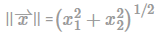For orthogonal matrix P,

PPT = PTP = 1

P2 × 2 &2x1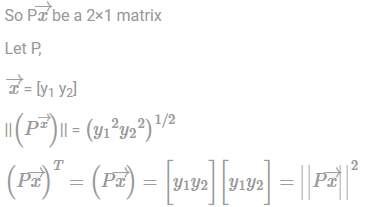hence,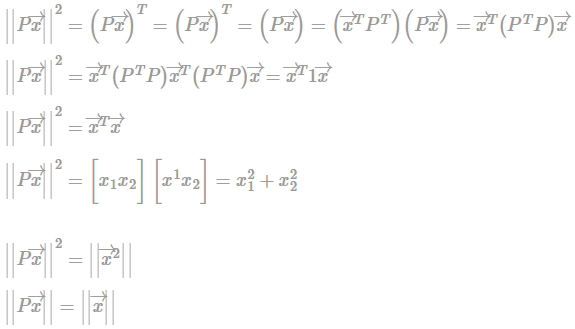Practice Test: Civil Engineering (CE)- 13 - Question 39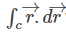where C is the curve x2 + y2 = 4

Detailed Solution for Practice Test: Civil Engineering (CE)- 13 - Question 39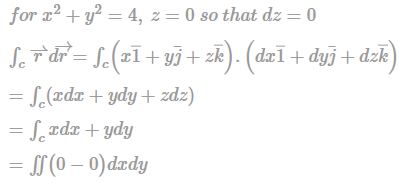(Using green theorem)

= 0

Practice Test: Civil Engineering (CE)- 13 - Question 40

Determine the specific gravity of the liquid Y for the fig. given below.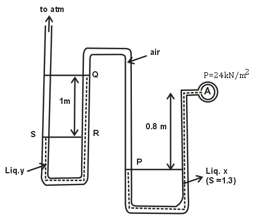Detailed Solution for Practice Test: Civil Engineering (CE)- 13 - Question 40

Given: SX = 1.3 pa = 24 k N/m2

As liquid has been moved towards A which means Pa will be vacuum pressure

pa = -24 kN/m2 Pressure at pt. P,

pP = -24 + (1.3 × 9.81 × 0.8) = -13.8 kN/m2 Air column is present between points P & Q;

Therefore pP = pQ

Also pt. S is at atm. Pressure, pS = 0 = pR

The specific gravity of liquid Y,

pS = pQ + (SY × 9.81 × 1)0

= -13.8 + (SY × 9.81 × 1) SY

= 1.406

Practice Test: Civil Engineering (CE)- 13 - Question 41

An ascending gradient of 1 in 50 meets a descending gradient of 1 in 40. The length of the vertical summit curve for a stopping sight distance of 200 m, will be ______m.

[Assume height of eye level of driver and object above roadway surface are 1.2 m and 0.18 m respectively]

Detailed Solution for Practice Test: Civil Engineering (CE)- 13 - Question 41

Stopping sight distance = 200 m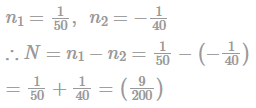∴ The length of summit vertical curve is given by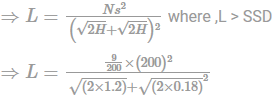= 389.69 m >200 m (O.K)

Practice Test: Civil Engineering (CE)- 13 - Question 42

The variance of the random variable X with probability density function f(x) = 1/2 |x|e-|x| is ___________.

Detailed Solution for Practice Test: Civil Engineering (CE)- 13 - Question 42

Given, f(x) = 1/2 |x|e-|x|

By definition, variance is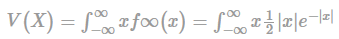dx since it is an odd function, we get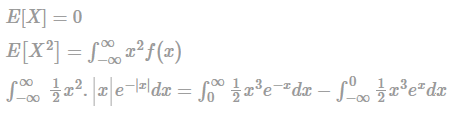Hence,

V(x) = 6 - (0)2

= 6.

Practice Test: Civil Engineering (CE)- 13 - Question 43

Let X and Y be independent random variables. Given that X has a uniform distribution over –1 ≤ x ≤ 1 and ▁Y = 2 and ▁(Y^2 )=6 Another random process W(t) is defined as W(t) = (Y + 3Xt) ⋅t

The mean square value of W(t) is

Detailed Solution for Practice Test: Civil Engineering (CE)- 13 - Question 43

Given, W(t) = (Y+3Xt).tE[W2(t)] = E[Y2 + 9 × 2t2 + 6 × Yt]t2 = E[Y2t2] + E[9 × 2t4] + E[XYt3] ∵ Y2 = 6 and X Y are independent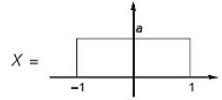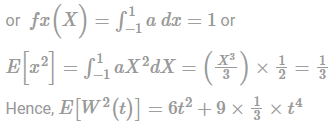Practice Test: Civil Engineering (CE)- 13 - Question 44

The state of the soil when plants fail to extract sufficient water for their requirements is _____.

Detailed Solution for Practice Test: Civil Engineering (CE)- 13 - Question 44

Permanent wilting point is defined as when a plant fails to extract sufficient water to grow crops. And finally the plant wilt up.

Practice Test: Civil Engineering (CE)- 13 - Question 45

The maximum shear stress in a rectangular beam is_____ times of average shear stress

Detailed Solution for Practice Test: Civil Engineering (CE)- 13 - Question 45

For Rectangular Sections.

For a rectangular section at any distance y from the Neutral Axis: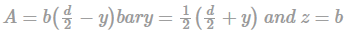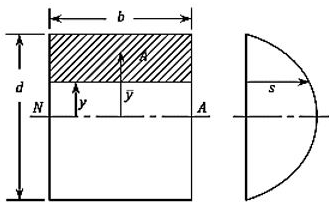Substituting in equation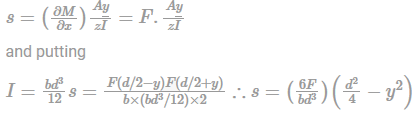This shows that there is a parabolic variation of Shear Stress with y. The maximum Shear Force occurs at the Neutral Axis and is given by:

s = 2F/2bd

If F/bd is called the Mean Stress then:

s = 1.5 × smean

Practice Test: Civil Engineering (CE)- 13 - Question 46

The laboratory tests on a soil sample gave the following results:

1). Natural water content, wn = 25%

2). Liquid limit, wL = 60%

3). Plastic limit, wp = 35%

4). Percentage of particle less than 2μ = 20%

Q. The value of activity number is

Detailed Solution for Practice Test: Civil Engineering (CE)- 13 - Question 46

Plasticity index,

Ip = WL-WP = 60-35 = 25%

Soil activity (A) is a number equal to IP/CF, where IP is the plasticity index of soil, and the CF is percentage of clay particles in soil (particles smaller than 2 microns)

Activity number

IP/c = 0.25/0.20 = 1.25

Practice Test: Civil Engineering (CE)- 13 - Question 47

An average data for conventional activated sludge treatment plant is as follows:

Wastewater flow = 35000 m3/d

Volume of aeration tank = 10500 m3

Influent BOD = 230 mg/L

Effluent BOD = 20 mg/L

Mixed Liquor suspended solids (MLSS) = 2200 mg/L

Effluent suspended solids = 30 mg/L

Waste sludge suspended solids = 9500 mg/L

Quantity of waste sludge = 200 m3/day

Assume MLVSS = 75% MLSS

Based on the above information, the oxygen requirement of the aeration tank is _________ tonnes/day.

Detailed Solution for Practice Test: Civil Engineering (CE)- 13 - Question 47

Given, V = 10500 m3, X = 2200 mg/L

Qo = 35000 m3/d; Qw = 200 m3/d

Xu = 9500 mg/L; Xe = 30 mg/L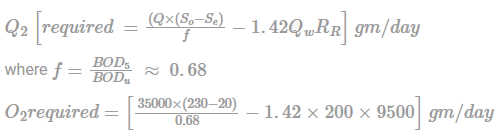O2 required = 8110823.53 gm/day

O2 required = 8.11 tonnes/day

Practice Test: Civil Engineering (CE)- 13 - Question 48

The Atterberg limits of a soil are LL = 68% and PL = 34% and it contains 85% by weight of clay. The water content of a sample is 45%. Determine the predominant clay mineral.

Detailed Solution for Practice Test: Civil Engineering (CE)- 13 - Question 48

Plasticity index IP = LL – PL = 68 − 34 = 34

Liquidity index IL = (w-PL)/IP = (45-34)/34 = 0.32

Activity, A = IP/%clay particles = 3485 = 0.40

The clay is likely to be predominantly kaolinite.

Practice Test: Civil Engineering (CE)- 13 - Question 49

A rectangular column section of 250mm x 400mm is reinforced with five steel bars of grade Fe 500, each of 20mm diameters. Concrete mix is M30. Axial load on the column section with minimum eccentricity as per IS: 456-2000 using limit state method can be applied upto

Detailed Solution for Practice Test: Civil Engineering (CE)- 13 - Question 49

A = 250 × 400 = 105 mm2

ASC = 5 × π/4(20)2 = 1570.8mm2

Ac = A−As = 98429.2mm2

Axial load, Pn = 0.4fckAc + 0.67 fy . Asc

= 0.4 × 30 × 98429.2 + 0.67 × 500 × 1570.8

= 1707.37 kN

Practice Test: Civil Engineering (CE)- 13 - Question 50

A prestressed concrete beam (pretensioned) has the following cross-section as shown in figure below. Initial prestress = 1200 N/mm2 (in both wires).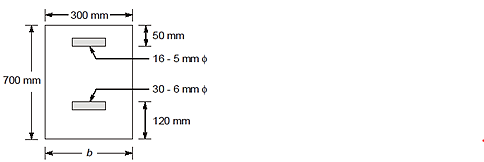Young’s modulus of steel, ES = 210 × 103 N/mm2, Young’s modulus of concrete, EC = 36 × 103 N/mm2

What will be the loss of pre-stress (in N/mm2) in top steel wires due to elastic shortening?

[Assume moment of Inertia about centroid axis = 8575 × 106 mm4]

Detailed Solution for Practice Test: Civil Engineering (CE)- 13 - Question 50

Resultant prestressing force

where, PR = Pt + PB ...(i)

Force in upper tensioned steel,

Pt = 16 × π/4 × 52 × 1200

= 376991 N = 376.991 kN

Force in bottom tensioned steel,

Ps = 30 × π/4 × 62 ×1200

= 1017876 N = 1017.876 kN

PR = 376991 + 1017876

= 1394867 N

Depth of centroidal axis from top,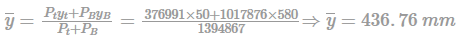Eccentricity of resultant force from the centre of beam section,

eR = 436.76 — 350 = 86.76 mm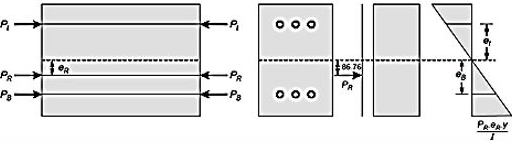Stress in concrete at the level of top steel,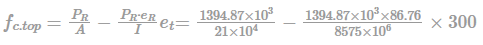loss of stress in top steel m.fc.top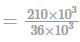= × 2.41 = 14.06N/mm2

*Answer can only contain numeric values
Practice Test: Civil Engineering (CE)- 13 - Question 51

A 60 mm-diameter water jet strikes a plate containing a hole of 40 mm diameter as shown in the figure. Part of the jet passes through the hole horizontally, and the remaining is deflected vertically. The density of water is 1000 kg/m3. If velocities are as indicated in the figure, the magnitude of horizontal force (in N) required to hold the plate is ______.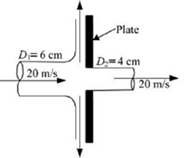Detailed Solution for Practice Test: Civil Engineering (CE)- 13 - Question 51
Force in X-direction=Rate of change of momentum

= (P1-Pt)x-direction

= m1V1-m2V2)x-direction

= VpA1V1 - pA2V2 (∴V1 = V2)

= pV2(A1-A2)

= 100 × 20 × 20 × π/4[0.06)2 - (0.04)2

= 628.32N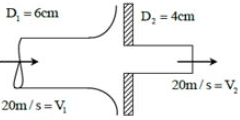Practice Test: Civil Engineering (CE)- 13 - Question 52

For a fluid the apparent viscosity is varying with rate of shear strain a shown in the following table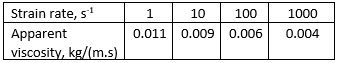Q. What type of fluid is

Detailed Solution for Practice Test: Civil Engineering (CE)- 13 - Question 52

As the rate of deformation increases, the apparent viscosity decreases. The behaviour of fluid is known as shear thinning which is also known as pseudoplastic fluid.

Practice Test: Civil Engineering (CE)- 13 - Question 53

Maximum strain theory for the failure of a material at the elastic limit is known as

Detailed Solution for Practice Test: Civil Engineering (CE)- 13 - Question 53

St. Venant's theory is also known as maximum principal strain theory.

According to this theory, material subjected to complex stresses fails in simple tensile tests, when maximum principal strain reaches the value of strain at yield point.

Practice Test: Civil Engineering (CE)- 13 - Question 54

For three soil layers A, B and C of cross-section 100mm x 100mm each shown, the permeability is 1.1 x 10-2 cm/sec, 2.9 x 10-3 cm/sec and 4.2 x 10-4 cm/sec respectively. The rate of water flow (in cm3/sec ) is

Detailed Solution for Practice Test: Civil Engineering (CE)- 13 - Question 54

Though it looks like horizontal flow, in actual condition it is vertical flow of water as there is no cross-section through each layer. Therefore, every layer has same flow q = q1 = q2 = q3 and the total head is ∆h = ∆h1 + ∆h2 + ∆h3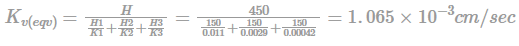Flow through soil, q = kv(eqv) ∆h/L A = 1.065 × 10-3cm/sec × 300/450 × (10cm × 10cm)

q = 0.071cm3/sec

Practice Test: Civil Engineering (CE)- 13 - Question 55

It required a time of 3 min 30 sec for 40% consolidation of a 35-mm-thick clay layer (2- way drainage condition) in the laboratory. How long (in days) will it take for a 4-m-thick clay layer of the same clay in the field under the same pressure increment to reach 40% consolidation? In the site, there is a rock layer at the bottom of the clay.

Detailed Solution for Practice Test: Civil Engineering (CE)- 13 - Question 55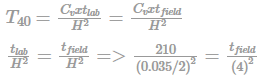12000 × 16 = tfield

⟹ 192000 sec = 133 days

Practice Test: Civil Engineering (CE)- 13 - Question 56

A sheet piling system with its corresponding flow net is shown in figure. Take and hydraulic conductivity as 2 x 10-5 cm/s. Estimate (a) rate flow in cm3/s (b) For the element A with l= 1.2m, calculate the average velocity.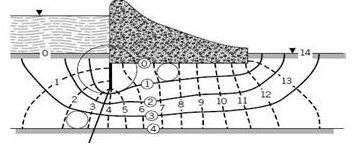Detailed Solution for Practice Test: Civil Engineering (CE)- 13 - Question 56

∆H =10 m

Here Nd= 14, Nf= 4

(a) q = k ∆H Nf/Nd

On substituting, flow rate, q = 2 x 10-5 x 10 x 102 x 4/14

q = 7.14 x 10-3 cm3/s = 0.0071 cm3/s

(b) velocity , v = ki

i = ∆H/L

i = 10/1.2 = 8.33

v = 2 x 10-5 x 8.33 = 1.67 x 10-4 cm/s = 0.00017 cm/s

*Answer can only contain numeric values
Practice Test: Civil Engineering (CE)- 13 - Question 57

A reservoir of surface area 400 hectares has water temperature = 27°C, relative humidity = 40%, ea of water = 17.54 mm (Hg) and velocity of wind 2 m above the ground is 11 km/hr. Use Meyer’s formula to determine the daily evaporation from the reservoir in mm.

Detailed Solution for Practice Test: Civil Engineering (CE)- 13 - Question 57
Evaporation using Meyer’s Formula

E = Km(es – ea) (1 + v9/16

Km = 0.36

Relative humidity, ea/es = 0.4

es = 17.54/0.4 = 43.85 mm of Hg

Velocity of wind at 9 m above ground, v9/v2 = (9/2)1/7

v9 = 11 × 1.239 = 13.629 km/hr

Putting in Meyer’s Formula, E = 0.36(43.85 – 17.54) (1 + 13.629/16) = 17.539 mm/day

Practice Test: Civil Engineering (CE)- 13 - Question 58

The following figure shows the velocity-time plot for a particle travelling along a straight line. The distance covered by the particle from t=0 to t=5s is ______ m.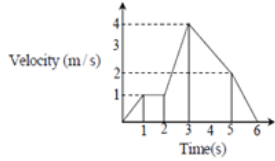Detailed Solution for Practice Test: Civil Engineering (CE)- 13 - Question 58
Area under V - T wave

Distance covered=Area under the curve between t = 0s to t = 5s

= 1/2 × 1 × 1 + 1 × 1 + 1/2 × (1 + 4) × (3 - 2) + 1/2 × (4 + 2) × (5-3)

= 0.5 + 1 + 2.5 + 6 = 10

Practice Test: Civil Engineering (CE)- 13 - Question 59

A bracket connection is formed as shown in figure using 4 bolts of 20 mm dia and grade 4.6. The service load P (in kN) that can be carried by the connection is

[Use LSM and assume failure to be critical in shear and failure shear plane lies in threaded portion of bolt]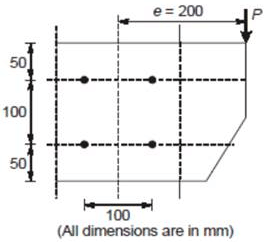Detailed Solution for Practice Test: Civil Engineering (CE)- 13 - Question 59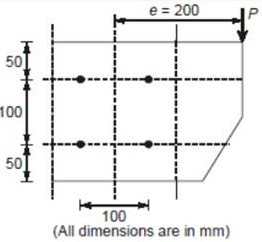Strength of bolt in single shear,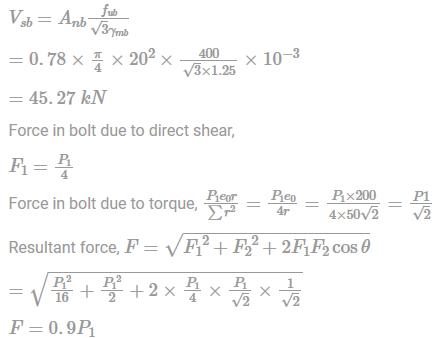For safety, F ≤ Vsb

0.9P1 = 45.27

P1 = 50.30 kN

P = P1/Load factor = 50.3/1.5 = 33.53 kN

Practice Test: Civil Engineering (CE)- 13 - Question 60

In a pair of overlapping photographs, the mean distance between two principal points both of which lie on the datum is 6.375 cm. At the time of photography, the aircraft was 600 m above the datum. The camera has a focal length of 150 mm. in the common overlap a tall chimney 120 m high with its base in the datum surface is observed. Determine the difference of parallax for top and bottom of chimney.

Detailed Solution for Practice Test: Civil Engineering (CE)- 13 - Question 60

Scale of the photograph for datum elevation,

S = f/H = (150/1000)/600

= 1/4000

For the datum elevation,

B/b = H/f

Or B = H/f . b

= 4000×6.375/100 = 255 m

The parallax for the top and bottom of the chimney are calculated from the equation

P = Bf / (H-h)

For bottom of chimney, h = 0

Hence p1 = 255×150/600 = 63.75 mm

For the top of chimney, h = 120 m

Hence p2 = 255×150 / (600-120)

= 79.69 mm

Difference of parallax is given by,

Δp = p2 – p1

= 79.69 – 63.75 = 15.94 mm.

Practice Test: Civil Engineering (CE)- 13 - Question 61

Solution of dy/dx = y2 × x3 at x = √5 and y = 2 is

Detailed Solution for Practice Test: Civil Engineering (CE)- 13 - Question 61

dy/dx = y2 × x3

⇒ dyy2 = x3dx

Integrating both sides.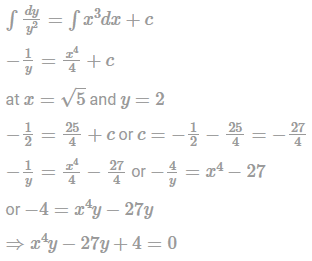*Answer can only contain numeric values
Practice Test: Civil Engineering (CE)- 13 - Question 62

The value of integral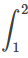exdx calculated using simpson's 1/3rd Rule, with 10 subintervals is use the table given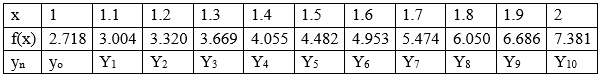Detailed Solution for Practice Test: Civil Engineering (CE)- 13 - Question 62
According to simpson’s 1/3rd Rule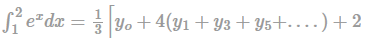y2 + y2 + y6 +...+ yn]

= 1/3 × 0.1[y0 + 4y1 + y3+ y5 + y7 + y9 +2

y2 + y4 + y6 + y8 + y10]

= 1/3 × 0.1[2.718+4(3.004+3.669+4.482

+5.474+6.686)+23.320+4.055+4.953+6.050+7.389]

0.1/3[2.718+423.315+218.378+7.389)

= 0.1/3[140.1232]exdx = 4.67077

*Answer can only contain numeric values
Practice Test: Civil Engineering (CE)- 13 - Question 63

In the following table, x is a discrete random variable and p(x) is the probability density. The difference between the variance & the standard deviation is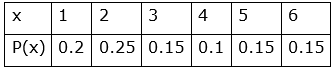Detailed Solution for Practice Test: Civil Engineering (CE)- 13 - Question 63
Ex = ∑ixipi = 1×2+2×0.25+3×0.15

+4×0.1×5×0.15+6×0.15

= 0.2+0.5+0.45+0.4+0.75+0.9 = 3.2

Ex2 = ∑ixi2pi = (1)2 × 0.2+(2)2×0.25

+(3)2×0.15+(4)2×0.1

+(5)2×0.15+(6)2×0.15

=1×0.2+4×0.25+9×0.15

+16×0.1+25×0.15+36×0.15

= 0.2+1+1.35+1.6+3.75+5.4=13.3

= Ex2-Ex2

Variance = 13.3-(3.2)2=13.3-10.24=3.06

Standard Deviation σ=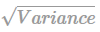== 1.749

Difference of variance & standard deviation = 3.06 – 1.749 = 1.311

Practice Test: Civil Engineering (CE)- 13 - Question 64

A fair coin is tossed independently five times. The probability of the event "the number of times tails show up is less than the number of times heads show up” is

Detailed Solution for Practice Test: Civil Engineering (CE)- 13 - Question 64

Coin is tossed 5 times

P(number of tails < number="" of="" />

= p5x & oT or 4x & IT or 3x & 2T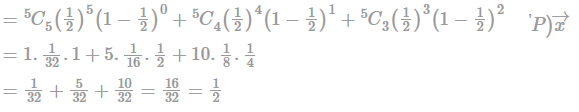Practice Test: Civil Engineering (CE)- 13 - Question 65

Consider the following equations

3x – y – z + 2w = 0

3x + y – z - 2w = 0

12x + 3y – 4z - 6w = 0

The system of equations will be

Detailed Solution for Practice Test: Civil Engineering (CE)- 13 - Question 65

The above system of equations can be written as AX = B.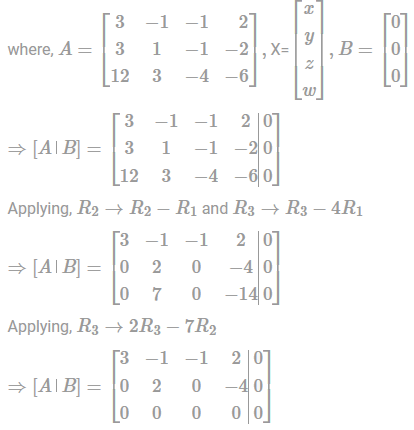∴ Rank (A) = Rank 2 and number of variables = 4

∴ The system is consistent having many solutions.

## GATE Civil Engineering (CE) 2023 Mock Test Series

27 docs|296 tests
Information about Practice Test: Civil Engineering (CE)- 13 Page
In this test you can find the Exam questions for Practice Test: Civil Engineering (CE)- 13 solved & explained in the simplest way possible. Besides giving Questions and answers for Practice Test: Civil Engineering (CE)- 13, EduRev gives you an ample number of Online tests for practice

## GATE Civil Engineering (CE) 2023 Mock Test Series

27 docs|296 tests(Scan QR code)